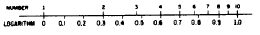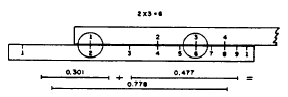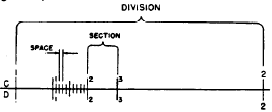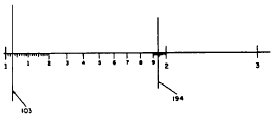Custom SearchTHE SLIDE RULE In 1620, not long after the invention of logarithms, Edmond Gunter showed how logarithmic calculations could be carried out mechanically. This is done by laying off lengths on a rule, representing the logarithms of numbers, and by combining these lengths in various ways. The idea was developed and with the contributions of Mannheim in 1851 the slide rule came into being as we know it today. The slide rule is a mechanical device by which we can carry out any arithmetic calculation with the exception of addition and subtraction. The most common operations with the slide rule are multiplication, division, finding the square or cube of a number, and finding the square root or cube root of a number. Also trigonometric operations are frequently performed. The advantage of the slide rule is that it can be used with relative ease to solve complicated problems. One limitation is that it will give results with a maximum of only three accurate significant digits. This is sufficient in most calculations, however, since most physical constants are only correct to two or three significant digits. When greater accuracy is required, other methods must be used. A simplified diagram of a slide rule is pictured in figure 8- 1. The sliding, central part of the rule is called the SLIDE. The movable glass or plastic runner with a hairline imprinted on it is called the INDICATOR. There is a C scale printed on the slide, and a D scale exactly the same as the C scale printed on the BODY or STOCK of the slide rule. The mark that is associated with the primary number 1 on any slide rule scale is called the INDEX. There isFigure 8-1.-Simplified diagram of a slide rule an index at the extreme left and at the extreme right on both the C and D scales. There are other scales, each having a particular use. Some of these will be mentioned later. SLIDE RULE THEORY We have mentioned that the slide rule is based on logarithms. Recall that, to multiply two numbers, we simply add their logarithms. Previously we found these logarithms in tables, but if the logarithms are laid off on scales such as the C and D scale of the slide rule, we can add the lengths, which represent these logarithms. To make such a scale we could mark off mantissas ranging from 0 to 1 on a rule as in figure 8-2. We then find in the tables the logarithms for numbers ranging from 1 to 10 and write the number opposite its corresponding logarithm on the scale.Figure 8-2.-Logarithms and corresponding numbers on a scale. Table 8-7 lists the numbers 1 through 10 and their corresponding logarithms to three places. These numbers are written opposite their logarithms on the scale shown in figure 8-2. If we have two such scales, exactly alike, arranged so that one of them is free to slide along the other, we can perform the operation of multiplication, for example, by ADDING LENGTHS; that is, by adding logarithms. For example, if we wish to multiply 2 x 3, we find the logarithm of 2 on the stationary scale and move the sliding scale so that its index is over that mark. We then add the logarithm of 3 by finding that logarithm on the sliding scale and by reading below it, on the stationary scale, the logarithm that is the sum of the two. Since we are not interested in the logarithms themselves, but rather in the numbers they represent, it is possible to remove the logarithmic notation on the scale in figure 8-2, and leave only the logarithmically spaced number scale. The C and D scales of the ordinary slide rule are made up in this manner. Figure 8-3 shows the multiplication of 2 x 3. Although the logarithm scales have been removed, the numbers 2 and 3 in reality signify the logarithms of Table 8-1.-Numbers and their corresponding logarithms.Figure 8-3.-Multiplication by use of the slide rule. 2 and 3, namely, 0.301 and 0.477; the product 6 on the scale really signifies the logarithm of 6, that is, 0.778. Thus, although logarithms are the underlying principle, we are able to work with the numbers directly. It should be noted that the scale is made up from mantissas only. The characteristic must be determined separately as in the case where tables are used. Since mantissas identify only the digit sequence, the digit 3 on the slide rule represents not only 3 but 30, 300, 0.003, 0.3, and so forth. Thus, the divisions may represent the number multiplied or divided by any power of 10. This is true also for numbers that fall between the divisions. The digit sequence, 1001, could represent 100.1, 1.001, 0.01001, and so forth. The following example shows the use of the same set of mantissas which appear in the foregoing example, but with a different characteristic and, therefore, a different answer: EXAMPLE: Use logs (positions on the slide rule) to multiply 20 times 30. SOLUTION:     log 20 = 1.301 (2 on the slide rule)     log 30 = 1.477 (3 on the slide rule)     __________________________     log of answer = 2.778 (6 on the slide rule) Since the 2 in the log of the answer is merely the indicator of the position of the decimal point in the answer itself, we do not expect to find it on the slide rule scale. As in the foregoing example, we find the digit 6 opposite the multiplier 3. This time, however, the 6 represents 600, because the characteristic of the log represented by 6 in this problem is 2. READING THE SCALES Reading a slide rule is no more complicated than reading a yard stick or ruler, if the differences in its markings are understood. Between the two indices of the C or D scales (the large digit 1 at the extreme left and right of the scales) are divisions numbered 2, 3, 4,5, 6, 7,8, and 9. Each length between two consecutive divisions is divided into 10 sections and each section is divided into spaces. (See fig- 8-4,)Figure 8-4.-Division, section, and space of a slide rule scale. Notice that the division between 1 and 2 occupies about one-third of the length of the rule. This is sufficient space in which to write a number for each of the section marks. The sections in the remaining divisions are not numbered, because the space is more limited. Notice also that in the division between 1 and 2, the sections are each divided into 10 spaces. The sections of the divisions from 2 to 4 are subdivided into only 5 spaces, and those from 4 to the right index are subdivided into only 2 spaces. These subdivisions are so arranged because of the limits of space. Only the sequence of significant digits is read on the slide rule. The position of the decimal point is determined separately. For example, if the hairline of the indicator is in the left-hand position shown in figure 8-5, the significant digits are read as follows:Figure 8-5.-Readings in the first division of a slide rule. 1. Any time the hairline falls in the first division, the first significant digit is 1. 2. Since the hairline lies between the index and the first section mark, we know the number lies between 1.0 and 1.1, or 10 and 11, or 100 and 110, etc. The second significant digit is 0. 3. We next find how far from the index the hairline is located. It lies on the marking for the third space. 4. The three significant digits are 103. In the second example shown in figure 8-5, the hairline is located in the first division, the ninth section, and on the fourth space mark of that section. Therefore, the significant digits are 194. number from 0 through 9 as its second digit and any number from 0 through 9 as its third digit. Sometimes a fourth digit can be roughly approximated in this first division, but the number is really accurate to only three significant digits. In the second and third divisions, each section is divided into only 5 spaces. (See fig. 8-6.) Thus, each space is equal to 0.2 of the section. Suppose, for example, that the hairline lies on the third space mark after the large 2 indicating the second division. The first significant digit is 2. Since the hairline lies between 2 and the first section mark, the second digit is 0. The hairline lies on the third space mark or 0.6 of the way between the division mark and the first section mark, so the third digit is 6.  Thus, the significant digits are 206. Notice that if the hairline lies on a space mark the third digit can be written accurately; otherwise it must be approximated. Thus, we see that any number falling in the first division of the slide rule will always have 1 as its first significant digit. It can have any From the fourth division to the right index, each section is divided into only .two spaces. Thus, if the hairline is in the fourth division and lies on the space mark between the sixth and seventh sections, we would read 465. If the hairline did not fall on a space mark, the third digit would have to be approximated.